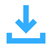Japanese Emoji Commands Mod 1.12.2. Hello all of you! Today I will introduce you a mod for Minecraft version 1.12.2 called Japanese Emoji Commands.Japanese Emoji Commands is a simple mod. It allows you to use (chat) Kaomoji easily in Minecraft. You won’t have to go online to find Kaomoji and then copy paste into Minecraft.

To use mod, you just need to type commands into chat. For example, if you want to have a smiley chat, you can write / happy and then press enter. On the chat line will appear (your name): (* ^ ω ^).Existing mod commands:

• /happy = (* ^ ω ^)
• /love = (❤ω❤)
• /blush = (ง ื▿ ื)ว
• /sympathy = (っ´ω`)ﾉ(╥ω╥)
• /notamused = (￣︿￣)
• /angry = ୧((#Φ益Φ#))୨
• /sad = ( ; ω ; )
• /scared = {{ (>_<) }}
• /indifferent = ╮( ˘ ､ ˘ )╭
• /confused = (＠_＠)
• /doubt = (￢_￢)
• /surprised = (o_O)
• /wave = (⌒ω⌒)ﾉ
• /hug = (つ✧ω✧)つ
• /wink = (^_~)
• /sorry = (* )人
• /nosebleed = (*￣ii￣)
• /hiding = ┬┴┬┴┤(･_├┬┴┬┴
• /writing = ( ￣ー￣)φ__
• /runs = ε=ε=┌( >_<)┘
• /sleep = (＿ ＿*) Z z z
• /cat = (=^-ω-^=)
• /bear = (￣(ｴ)￣)
• /dog = U^ｪ^U
• /rabbit = ／(^ x ^)＼
• /pig = ｡ﾟ(ﾟ´(00)`ﾟ)ﾟ｡
• /bird = (◉Θ◉)
• /fish = (°)#))<<
• /spider = /╲/╭[ ᴼᴼ ౪ ᴼᴼ]╮/╱\
• /friends = ヽ( ⌒ω⌒)人(=^‥^= )ﾉ
• /enemies = /( .□.)＼ ︵╰(°益°)╯︵ /(.□. /)
• /weapons = (ಠ o ಠ)¤=[]:::::>
• /magic = (ﾉ>ω<)ﾉ :｡･::･ﾟ’★,｡･::･ﾟ’☆
• /food = ( ˘▽˘)っ♨
• /music = ♪♬((d⌒ω⌒b))♬♪
• /unflip = ┬─┬ ノ( ゜-゜ノ)
• /tableflip = (╯°□°）╯︵ ┻━┻
• /shrug = ¯\_(ツ)_/¯Requirement:>  for Minecraft 1.12.2